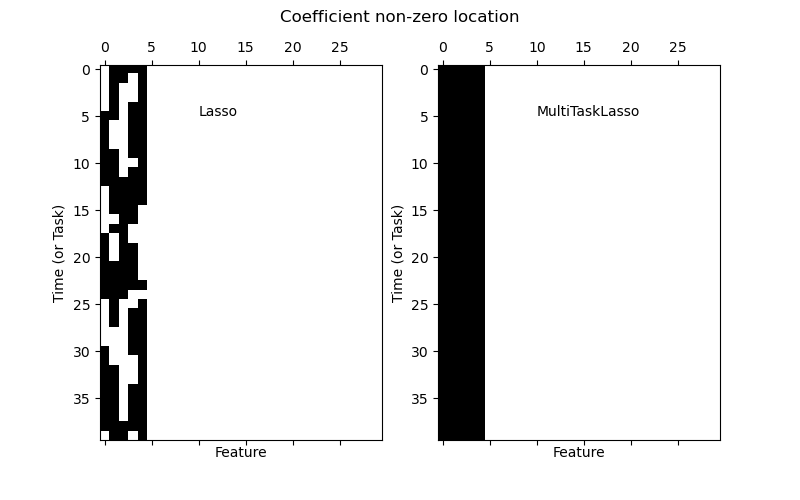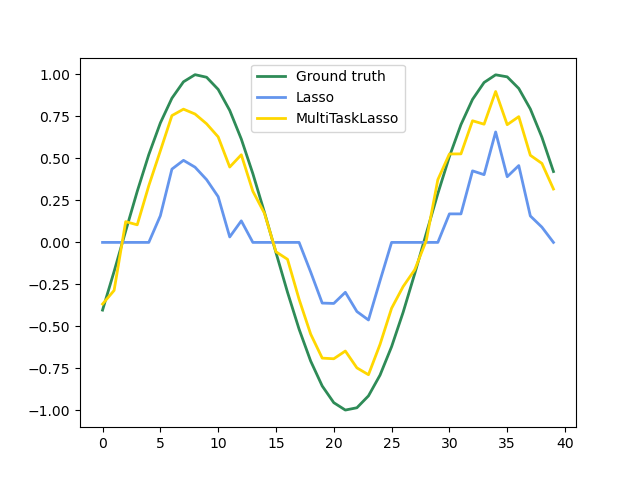# Joint feature selection with multi-task Lasso¶

The multi-task lasso allows to fit multiple regression problems jointly enforcing the selected features to be the same across tasks. This example simulates sequential measurements, each task is a time instant, and the relevant features vary in amplitude over time while being the same. The multi-task lasso imposes that features that are selected at one time point are select for all time point. This makes feature selection by the Lasso more stable.

••print(__doc__)

# Author: Alexandre Gramfort <alexandre.gramfort@inria.fr>

import matplotlib.pyplot as plt
import numpy as np

rng = np.random.RandomState(42)

# Generate some 2D coefficients with sine waves with random frequency and phase
n_samples, n_features, n_tasks = 100, 30, 40
n_relevant_features = 5
times = np.linspace(0, 2 * np.pi, n_tasks)
for k in range(n_relevant_features):
coef[:, k] = np.sin((1. + rng.randn(1)) * times + 3 * rng.randn(1))

X = rng.randn(n_samples, n_features)
Y = np.dot(X, coef.T) + rng.randn(n_samples, n_tasks)

coef_lasso_ = np.array([Lasso(alpha=0.5).fit(X, y).coef_ for y in Y.T])

# #############################################################################
# Plot support and time series
fig = plt.figure(figsize=(8, 5))
plt.subplot(1, 2, 1)
plt.spy(coef_lasso_)
plt.xlabel('Feature')
plt.text(10, 5, 'Lasso')
plt.subplot(1, 2, 2)
plt.xlabel('Feature')
fig.suptitle('Coefficient non-zero location')

feature_to_plot = 0
plt.figure()
lw = 2
plt.plot(coef[:, feature_to_plot], color='seagreen', linewidth=lw,
label='Ground truth')
plt.plot(coef_lasso_[:, feature_to_plot], color='cornflowerblue', linewidth=lw,
label='Lasso')